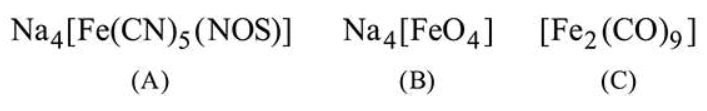# The oxidation states of iron atoms in compounds

Question:

The oxidation states of iron atoms in compounds (A), (B) and $(\mathrm{C})$, respectively, are $x, y$ and $z$. The sum of $x, y$ and $z$ is _________.Solution:

(6)

The oxidation states of iron in these compounds will be -

$\ln A, x+5(-1)+(-1)=-4 \Rightarrow x=+2$

In B, $y+4(-2)=-4 \Rightarrow y=+4$

In $\mathrm{C}, z=0$

The sum of oxidation states will be $=4+2+0=6$.